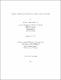## Groups with condition on non-permutable subgroups2015
##### Publisher
University of Alabama Libraries
##### Abstract

In this dissertation, we study the structure of groups satisfying the weak minimal condition and weak maximal condition on non-permutable subgroups. In Chapter 1, we discuss some definitions and well-known results that we will be using during the dissertation. In Chapter 2, we establish some preliminary results which will be useful during the proof of the main results. In Chapter 3, we express our main results, one of which states that a locally finite group satisfying the weak minimal condition on non-permutable subgroups is either Chernikov or quasihamiltonian. We also prove that, a generalized radical group satisfying the weak minimal condition on non-permutable subgroups is either Chernikov or is soluble-by-finite of finite rank. In the Final Chapter, we will discuss the class of groups satisfying the weak maximal condition on non-permutable subgroups.

##### Description
Electronic Thesis or Dissertation
Mathematics